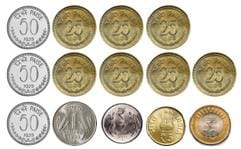Olympiad Test Level 2: Numbers’ Magic- 1

# Olympiad Test Level 2: Numbers’ Magic- 1 - Class 3

Test Description

## 10 Questions MCQ Test GK Olympiad for Class 3 - Olympiad Test Level 2: Numbers’ Magic- 1

Olympiad Test Level 2: Numbers’ Magic- 1 for Class 3 2023 is part of GK Olympiad for Class 3 preparation. The Olympiad Test Level 2: Numbers’ Magic- 1 questions and answers have been prepared according to the Class 3 exam syllabus.The Olympiad Test Level 2: Numbers’ Magic- 1 MCQs are made for Class 3 2023 Exam. Find important definitions, questions, notes, meanings, examples, exercises, MCQs and online tests for Olympiad Test Level 2: Numbers’ Magic- 1 below.
 1 Crore+ students have signed up on EduRev. Have you?
Olympiad Test Level 2: Numbers’ Magic- 1 - Question 1

### What number should be added to 5.346 to make it a double of 3.8?

Detailed Solution for Olympiad Test Level 2: Numbers’ Magic- 1 - Question 1

Double of 3.8 = 3.8 × 2 = 7.6 Number to be added = 7.6 – 5.346 = 2.254

Olympiad Test Level 2: Numbers’ Magic- 1 - Question 2

### Manpreet purchased a moving dragon toy for Rs. 285/-. Which of the following options shows the correct amount of money as the cost of toy purchased?

Detailed Solution for Olympiad Test Level 2: Numbers’ Magic- 1 - Question 2

Rs. 200 + Rs. 50 + Rs. 20 + Rs. 10 + Rs. 5
= Rs. 285

Olympiad Test Level 2: Numbers’ Magic- 1 - Question 3

### Nine coins of Rs. 5 and three coins of Rs. 10 is equivalent to ____________.

Detailed Solution for Olympiad Test Level 2: Numbers’ Magic- 1 - Question 3

As (5 × 9 = 45) + (10 × 3 = 30) = 45 + 30 = 75

Olympiad Test Level 2: Numbers’ Magic- 1 - Question 4

There are 4,836 apples in the first orchard. In the second orchard, there are 2,147 less apples as compared to the first orchard. In the third orchard, the number of apples is 1,935 more than the second orchard.

How many apples are there in the second orchard?

Detailed Solution for Olympiad Test Level 2: Numbers’ Magic- 1 - Question 4

Apples in the second orchard
= 4836 – 2147 = 2689

Olympiad Test Level 2: Numbers’ Magic- 1 - Question 5

There are 4,836 apples in the first orchard. In the second orchard, there are 2,147 less apples as compared to the first orchard. In the third orchard, the number of apples is 1,935 more than the second orchard.

Which orchard has maximum number of apples?

Detailed Solution for Olympiad Test Level 2: Numbers’ Magic- 1 - Question 5

As 4836 > 4624 > 2689, so, the first orchard has maximum apples.

Olympiad Test Level 2: Numbers’ Magic- 1 - Question 6

Find the difference between the greatest and the smallest numbers formed using the digits 4, 1, 8, 6?

Detailed Solution for Olympiad Test Level 2: Numbers’ Magic- 1 - Question 6

Greatest number that can be formed = 8641 Smallest number that can be formed = 1468 Difference = 8641 – 1468 = 7173

Olympiad Test Level 2: Numbers’ Magic- 1 - Question 7

Manan went to a toy shop and liked the following toys. He had Rs.130/- with him.
Bouncy Ball Rs. 30/-
Squeezy Duck Rs. 25/-
Push back Car Rs. 75/-
Train Rs. 80/-
Drum Rs. 70/-
Teddy Bear Rs. 95/-

Which of these items can Manan buy for himself?

Detailed Solution for Olympiad Test Level 2: Numbers’ Magic- 1 - Question 7

Total cost of toys
= Rs. 30 + Rs. 25 + Rs. 75 = Rs. 130

Olympiad Test Level 2: Numbers’ Magic- 1 - Question 8

Manan went to a toy shop and liked the following toys. He had Rs.130/- with him.
Bouncy Ball Rs. 30/-
Squeezy Duck Rs. 25/-
Push back Car Rs. 75/-
Train Rs. 80/-
Drum Rs. 70/-
Teddy Bear Rs. 95/-

How much more money is needed by Manan to buy Train and Teddy Bear together?

Detailed Solution for Olympiad Test Level 2: Numbers’ Magic- 1 - Question 8

Total cost of train and teddy bear = Rs. 80 + Rs. 95 = Rs. 175
More money needed
= Rs. 175 – Rs. 130 = Rs. 45

Olympiad Test Level 2: Numbers’ Magic- 1 - Question 9

What is the total value of coins shown here?Detailed Solution for Olympiad Test Level 2: Numbers’ Magic- 1 - Question 9

Rs. 10 + Rs. 5 + Rs. 2 + Rs. 1 = Rs. 18
(50 paise × 3) + (25 paise × 7)
= Rs. 1.50 + Rs. 1.75 = Rs. 3.25
Total amount
= Rs. 18 + Rs. 3.25 = Rs. 21.25

Olympiad Test Level 2: Numbers’ Magic- 1 - Question 10

Read the following sentences and mark your responses as True and False. Then choose the correct option.
(A) A week has seven days.
(B) There are fifty seconds in one hour.
(C) The meal we take at 8 am is dinner.
(D) Quarter to 4 means 4:45.

Detailed Solution for Olympiad Test Level 2: Numbers’ Magic- 1 - Question 10

There are sixty seconds in one hour, the meal we take at 8 am is breakfast and at 8 pm is dinner, Quarter to 4 means 3:45.

48 tests